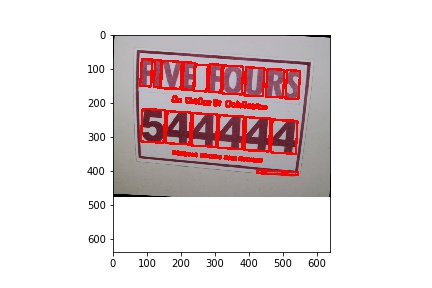# Fine-tuning the detector¶

This example shows how to fine-tune the recognizer using an existing dataset. In this case, we will use the text segmentation dataset from ICDAR 2013, available from https://rrc.cvc.uab.es/?ch=1&com=downloads.

First, we download our dataset. `keras-ocr` provides a convenience function for this, which you are welcome to examine to understand how the dataset is downloaded and parsed.

An interactive version of this example on Google Colab is provided here.

```data_dir = '.'

import os
import math
import imgaug
import numpy as np
import matplotlib.pyplot as plt
import sklearn.model_selection
import tensorflow as tf

import keras_ocr

dataset = keras_ocr.datasets.get_icdar_2013_detector_dataset(
cache_dir='.',
skip_illegible=False
)
```

Now we split the dataset into training and validation.

```train, validation = sklearn.model_selection.train_test_split(
dataset, train_size=0.8, random_state=42
)
augmenter = imgaug.augmenters.Sequential([
imgaug.augmenters.Affine(
scale=(1.0, 1.2),
rotate=(-5, 5)
),
imgaug.augmenters.GaussianBlur(sigma=(0, 0.5)),
imgaug.augmenters.Multiply((0.8, 1.2), per_channel=0.2)
])
generator_kwargs = {'width': 640, 'height': 640}
training_image_generator = keras_ocr.datasets.get_detector_image_generator(
labels=train,
augmenter=augmenter,
**generator_kwargs
)
validation_image_generator = keras_ocr.datasets.get_detector_image_generator(
labels=validation,
**generator_kwargs
)
```

We can visualize what the samples look like pretty easily.

```image, lines, confidence = next(training_image_generator)
canvas = keras_ocr.tools.drawBoxes(image=image, boxes=lines, boxes_format='lines')
plt.imshow(canvas)
```Now we can build the detector and train it.

```detector = keras_ocr.detection.Detector()

batch_size = 1
training_generator, validation_generator = [
detector.get_batch_generator(
image_generator=image_generator, batch_size=batch_size
) for image_generator in
[training_image_generator, validation_image_generator]
]
detector.model.fit_generator(
generator=training_generator,
steps_per_epoch=math.ceil(len(train) / batch_size),
epochs=1000,
workers=0,
callbacks=[
tf.keras.callbacks.EarlyStopping(restore_best_weights=True, patience=5),
tf.keras.callbacks.CSVLogger(os.path.join(data_dir, 'detector_icdar2013.csv')),
tf.keras.callbacks.ModelCheckpoint(filepath=os.path.join(data_dir, 'detector_icdar2013.h5'))
],
validation_data=validation_generator,
validation_steps=math.ceil(len(validation) / batch_size)
)
```

Weights can be loaded into the model attribute of the detector. This is how you can reuse the weights later.

```detector.model.load_weights(os.path.join(data_dir, 'detector_icdar2013.h5'))
```